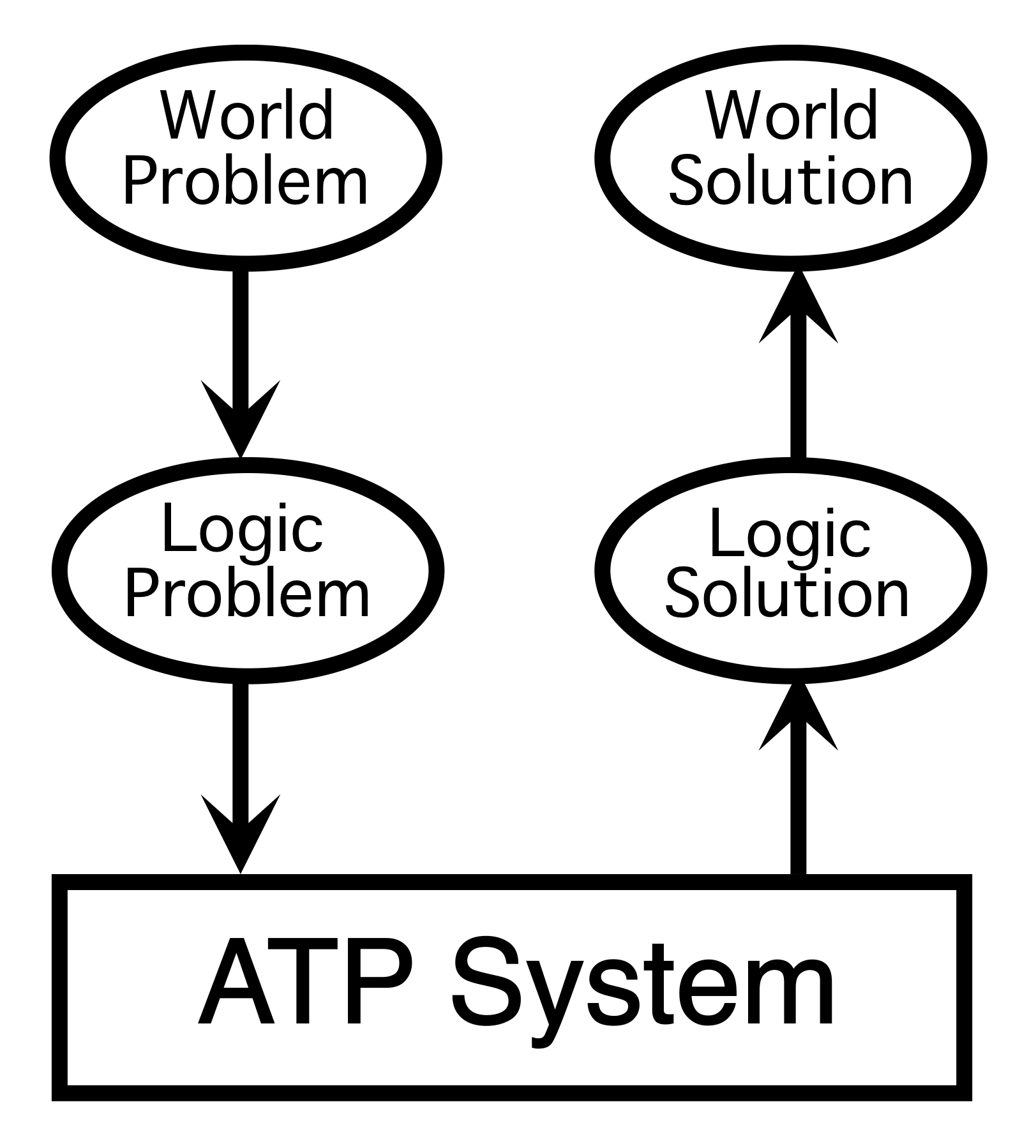# What is Automated Reasoning?### The World Has Problems

• Translate world problem to logic
• Axioms describe what you know about the world
• Conjecture describes what need to be solved
• Use Automated Reasoning to get a logic solution
• Theorem provers find proofs
• Model finders finite countermodels
• Model finders check axioms are consistent
• Translate logic solution to world
• Proof of the conjecture describes the solution
• Countermodel is an example of why there is no solution

### Automated Reasoning Systems

• Computer programs that (try to) determine if
• A conjecture C is a logical consequence of a set of axioms Ax
The derivation of conclusions that follow inevitably from facts.

• Computer programs that (try to) determine if
• A set of statements S is satisfiable
Establishing consistency and non-logical consequence